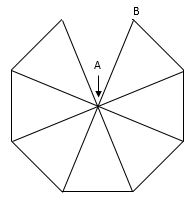# Triangle CircuitA circuit is built by connecting $1\ \Omega$ wires in a pattern of triangles, as shown above. Point $A$ is the common vertex of all triangles, and point $B$ is a vertex of one of the triangles at the end of the pattern.

Let $n$ be the number of triangles in the pattern. (In the diagram above, $n = 7$.)

Determine the resistance between points $A$ and $B$ in the limit where the pattern of wires grows to infinitely many triangles, i.e. $\lim_{n\to\infty} R_{AB}$

Give your answer to 3 decimal places.

×

Problem Loading...

Note Loading...

Set Loading...